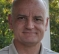## GFSK parametersStarted by 6 years ago4 replieslatest reply 6 years ago969 views

I'm trying to understand the exact relationship between the frequency deviation and the time-bandwidth product for GFSK modulation.

The basic set of parameters for GFSK include the bit/symbol rate (assuming 1 bit per symbol), BT, 3dB bandwidth and delta-f (the deviation from carrier frequency).

I sometimes see cases where several of these are defined simultaneously, but I think that may mean that they are over specified.

If I have the symbol rate and the BT product, isn't everything now specified?

[ - ]Hello dgshaw6,

The frequency deviation is error in center frequency, and function of HW implementation, wouldn't be related to BT, 3dB BW.

BT is related to 3dB BW in baseband, so perhaps restating it, implies the radio spectrum 3dB BW.

Best regards,

Shahram

[ - ]The frequency deviation I was defining is the deviation from the carrier for the mark and space frequencies, rather than the carrier offset frequency.

[ - ]What makes these calculation hard is that in FSK and its derivatives, the modulating signal is in the argument of a cosine - hence it is a highly non-linear function. I have designed a system like this and there probably is some formula for 3dB bandwidth calculation, but in practice that is not very relevant.

At least in my case the problem is more in the 'outskirts' of the signal - You usually have to obey some spectrum mask which is not only defined by a 3dB bandwidth, but also by allowing only so much 'bleeding' into neighbouring channels.

There are a few rules of thumb, nevertheless (and then you have to simulate to get more exact results):

- If the data rate as in symbols/second is a lot higher than the frequency deviation it becomes the dominat factor and the other way around.

- The frequency deviation has more impact on the shape of the spectrum close to the carrier and the symbol-rate has more effect on the shape far from the carrier. You can of course always increase the data-rate by using more bit/symbol - 4-FSK or more, but that doesn't really change the shape of the spectrum.

[ - ]